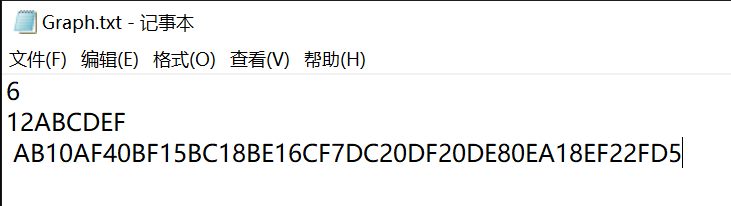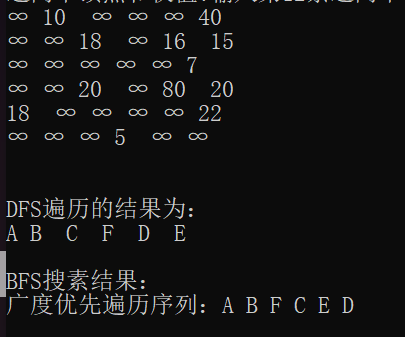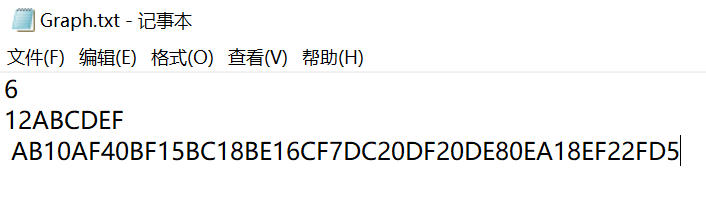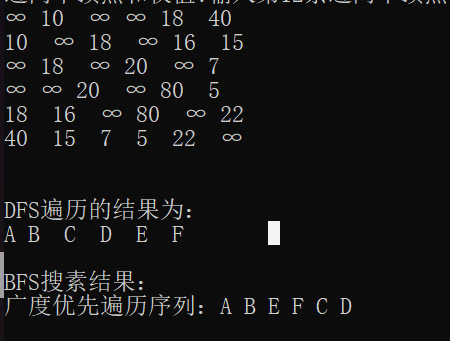• DFS、BFC算法说明代码实现测试用例 说明 BFS算法我们常用的方法就是...下面提供有向和无向图的DFS、BFS的实现 想要得到有向和无向只需要在void createMGraph函数里面修改即可，我也做了标记，供大家参考 代码实现 #de
DFS、BFC算法说明代码实现测试用例
说明
BFS算法我们常用的方法就是利用队列来处理，访问一个结点，就访问所有与它有关的结点，然后反复，比较简单
DFS算法便是访问一个结点，然后一直访问下去，直到没有结点可访问，可谓“不撞南墙不回头”，该算法最重要的就是实现int FirstAdjVex函数、int NextAdjVex函数这两个函数的实现
下面提供有向和无向图的DFS、BFS的实现
想要得到有向和无向只需要在void createMGraph函数里面修改即可，我也做了标记，供大家参考
代码实现
#define _CRT_SECURE_NO_WARNINGS
#include <stdio.h>
#include <stdlib.h>
#include <stdbool.h>
#include <limits.h>

#define MAX_VERTEX_NUM 20    //最大顶点个数
#define INFINITY 0x3f3f3f3f  //最大值∞

typedef char VertexType;   //顶点向量类型
typedef int VRType;
typedef int InfoType;
typedef int QElemType;

//图的数组存储表示
typedef struct {
VertexType vexs[MAX_VERTEX_NUM];    //顶点向量
VRType arcs[MAX_VERTEX_NUM][MAX_VERTEX_NUM];
//邻接矩阵,于无权图1、0表示两个顶点是否相邻，对于有权图为权值
int vexnum, arcnum; //图的顶点数和弧数
}MGraph;

bool visited[MAX_VERTEX_NUM];  //标记顶点是否被访问，访问为true,否则为false

//查找顶点在顶点向量中的位置
int locateVertex(MGraph umg, VertexType v)
{
int i;
for (i = 0; i < umg.vexnum; i++)
{
if (v == umg.vexs[i])
return i;
}
return -1;
}
//创建无向有权图
void createMGraph(FILE* fp, MGraph* mg)
{
int i, j, v, w;
char v1, v2;
printf("输入有权图的顶点数和边数，注意输入的第一个值将作为后序遍历的起始位置\n");
fscanf_s(fp, "%d%d", &(*mg).vexnum, &(*mg).arcnum);
for (v = 0; v < (*mg).vexnum; v++)//初始化
visited[v] = false;
printf("输入顶点名称\n");
for (v = 0; v < (*mg).vexnum; v++)
{
printf("输入第%d个顶点名称：", v);
fscanf_s(fp, "%c", &(*mg).vexs[v], 1);
//     getchar();
}
//初始化邻接矩阵
for (i = 0; i < (*mg).vexnum; i++)
for (j = 0; j < (*mg).vexnum; j++)
(*mg).arcs[i][j] = INFINITY;
//将图中相邻的顶点的邻接矩阵值设为边的权值
printf("输入边的信息，输入边的两个顶点名称和权值v1 v2 w\n");
for (v = 0; v < (*mg).arcnum + 1; v++)
{
printf("输入第%d条边两个顶点和权值:", v);
fscanf_s(fp, "%c", &v1, 1);
fscanf_s(fp, "%c", &v2, 1);
fscanf_s(fp, "%d", &w);
//  fscanf(fp,"%c%c%d",, &v2, &w);
// getchar();
i = locateVertex(*mg, v1);
j = locateVertex(*mg, v2);
==(*mg).arcs[i][j] = (*mg).arcs[j][i] = w;    //无向图，一条边关联两个顶点==
==// (*mg).arcs[i][j] = w;//有向图，边为单向==
}
}

//打印图的邻接矩阵
void print(MGraph G)
{
int i, j;
printf("图的邻接矩阵\n");
for (i = 0; i < G.vexnum; i++)
{
for (j = 0; j < G.vexnum; j++)
{
if (G.arcs[i][j] != INFINITY)
printf("%d  ", G.arcs[i][j]);
else
printf("∞ ");
}
printf("\n");
}
printf("\n");
}

//深度优先遍历图
int FirstAdjVex(MGraph G, int v)
{
//获取与顶点v相邻的第一个顶点下标
int i;
for (i = 0; i < G.vexnum; i++)
{
if (G.arcs[v][i] != 0 && G.arcs[v][i] != INFINITY && !visited[i])
return i;
}
return -1;
}

int NextAdjVex(MGraph G, int v, int w)
{   //得到v的下一个未被访问的相邻顶点下标
int i;
for (i = w; i < G.vexnum; i++)
{
if (G.arcs[v][i] != 0 && G.arcs[v][i] != INFINITY && !visited[i])
return i;
}
return -1;
}

void DFS(MGraph G, int v)
{
//请在此处填写代码实现深度优先遍历v顶点所在的连通分量
//初始化
visited[v] = true;
int w;
w = FirstAdjVex(G, v);
while (w != -1)
{
printf("%c  ", G.vexs[w]);
if (visited[w] != true)
DFS(G, w);
w = NextAdjVex(G, v, w);//更新w的值
}
}

void DFSTraverse(MGraph G)
{
printf("DFS遍历的结果为：\n");
printf("%c ", G.vexs);
DFS(G, 0);
printf("\n");
}

typedef struct QNode
{
QElemType data;
struct QNode* qnext;
}QNode, * PQNode;

typedef struct Queue
{
PQNode front;
PQNode rear;
}Queue, * PQueue;

//初始化一个空队列
PQueue initQueue()
{
PQueue pqueue = (PQueue)malloc(sizeof(Queue));
PQNode pqnode = (PQNode)malloc(sizeof(QNode));
if (pqnode == NULL)
{
printf("队列头空间申请失败！\n");
exit(-1);
}
if (pqueue && pqnode)
{
pqueue->front = pqueue->rear = pqnode;
pqnode->qnext = NULL;
return pqueue;
}
}

//队尾入队
void enQueue(PQueue pqueue, QElemType data)
{
PQNode pqnode = (PQNode)malloc(sizeof(QNode));
if (pqnode == NULL)
{
printf("队列结点申请失败！\n");
exit(-1);
}
pqnode->data = data;
pqnode->qnext = NULL;
pqueue->rear->qnext = pqnode;
pqueue->rear = pqnode;
}
//判断队列是否为空
bool isEmpty(PQueue pqueue)
{
if (pqueue->front == pqueue->rear)
return true;
return false;
}
//队头出队
QElemType deQueue(PQueue pqueue)
{
if (isEmpty(pqueue))
{
printf("队列为空\n");
exit(-1);
}
PQNode pqnode = pqueue->front->qnext;
pqueue->front->qnext = pqnode->qnext;
if (pqnode == pqueue->rear)
pqueue->rear = pqueue->front;
QElemType data = pqnode->data;
free(pqnode);
return data;

}
void BFSTraverse(MGraph G)
{
printf("广度优先遍历序列：");
int i, v, w;
for (i = 0; i < G.vexnum; i++)
visited[i] = false;
PQueue pqueue = initQueue();    //初始化辅助队列
for (i = 0; i < G.vexnum; i++)
{
if (!visited[i])             //i未被访问
{
visited[i] = true;
printf("%c ", G.vexs[i]);
enQueue(pqueue, i);
while (!isEmpty(pqueue))
{
v = deQueue(pqueue);    //队头元素出队
for (w = FirstAdjVex(G, v); w >= 0; w = NextAdjVex(G, v, w))
if (!visited[w])            //w为v的尚未访问的邻接顶点
{
visited[w] = true;
printf("%c ", G.vexs[w]);
enQueue(pqueue, w);
}
}
}
}
printf("\n");
}

int main()
{
printf("带权有向图测试：\n");
MGraph mg;
FILE* fp;
fp = fopen("Graph.txt", "r");
createMGraph(fp, &mg);
print(mg);
printf("\n");
DFSTraverse(mg);
printf("\n");
printf("BFS搜素结果：\n");
BFSTraverse(mg);
printf("\n");

return 0;
}

测试用例
有向图测试用例：无向图测试用例：展开全文数据结构 算法 队列 dfs
• 一个n行m列地图 地图上每个格子要么是o，要么是x o表示这个格子是安全 x表示这个格子不能进入 你现在在第0行，第0列（左上角） 你希望走到第x行，第y列 输入保证（0,0）是安全 向上走一格耗时a分钟 下走一...
/*
有一个n行m列的地图
地图上每个格子要么是o，要么是x
o表示这个格子是安全的
x表示这个格子不能进入
你现在在第0行，第0列（左上角）
你希望走到第x行，第y列
输入保证（0,0）是安全的
向上走一格耗时a分钟
向下走一格耗时b分钟
向左走一格耗时c分钟
向右走一格耗时d分钟
问走到目的地点（第x行，第y列）最快需要多少分钟
如果无法走到，输出-1
输入描述：
第一行输入T表示有T组数据（1<=T<=30）
每组数据
第一行输入两个整数 n，m（1 <= n, m <= 200）
第二行输入两个整数 x,y (0 <= x < n, 0 <= y < m)
第三行输入四个整数 a,b,c,d (0 <= a, b, c, d <= 300)
接下来n行每行m个字符('o’或者’x’表示地图)
输出描述：
对于第i组测试数据，输出一行
Case#i : ans
示例1：
2
5 5
2 2
1 5 25 125
ooooo
oxxxo
oxooo
oxxxo
ooooo
5 5
2 2
0 0 0 0
ooooo
oxxxo
oxoxo
oxxxo
ooooo
case #1: 560
case #2: -1
说明
560 = 125 * 4 + 5 * 2 + 25 * 2
*/
/*
思路：
利用BFS 搜索思想，从(0,0)点开始，每次搜索四周可以到达的结点，直到搜到终点， 在求最小权重的问题上（或者最小路径），第一个找到的解就是最小的权重（或者最小路径）（也只能找到一条路径,因为访问过的点已经做了标记不能再访问）
因为路径远的权重肯定大于路径的近的权重，当路径相等时，权重一定相等。
*/
/*
有一个n行m列的地图
地图上每个格子要么是o，要么是x
o表示这个格子是安全的
x表示这个格子不能进入

你现在在第0行，第0列（左上角）
你希望走到第x行，第y列

输入保证（0,0）是安全的

向上走一格耗时a分钟
向下走一格耗时b分钟
向左走一格耗时c分钟
向右走一格耗时d分钟

问走到目的地点（第x行，第y列）最快需要多少分钟

如果无法走到，输出-1

输入描述：
第一行输入T表示有T组数据（1<=T<=30）
每组数据
第一行输入两个整数 n，m（1 <= n, m <= 200）
第二行输入两个整数 x,y (0 <= x < n, 0 <= y < m)
第三行输入四个整数 a,b,c,d (0 <= a, b, c, d <= 300)
接下来n行每行m个字符('o'或者'x'表示地图)

输出描述：
对于第i组测试数据，输出一行
Case#i : ans

示例1：
2
5 5
2 2
1 5 25 125
ooooo
oxxxo
oxooo
oxxxo
ooooo
5 5
2 2
0 0 0 0
ooooo
oxxxo
oxoxo
oxxxo
ooooo

case #1: 560
case #2: -1

说明
560 = 125 * 4 + 5 * 2 + 25 * 2
*/

/*
思路：
利用BFS 搜索思想，从(0,0)点开始，每次搜索四周可以到达的结点，直到搜到终点， 在求最小权重的问题上（或者最小路径），第一个找到的解就是最小的权重（或者最小路径）（也只能找到一条路径,因为访问过的点已经做了标记不能再访问）
因为路径远的权重肯定大于路径的近的权重，当路径相等时，权重一定相等。
*/
#include<stdio.h>
#include<string.h>
#include<stdlib.h>
#include<iostream>
#include<queue>
#include<vector>
using namespace std;
#define MAXGRAPH 201
//vector<int> cost;
int n, m, x, y, a, b, c, d;
int visited[MAXGRAPH][MAXGRAPH];
char graph[MAXGRAPH][MAXGRAPH];

int move_x = { -1, 1, 0, 0 }; //移动x的方向
int move_y = { 0, 0, -1, 1 }; //移动y的方向
struct node
{
int x;
int y;
int minutes;
};

bool check(int x, int y)
{
if (x >= 0 && x < n)
{
if (y >= 0 && y < m)
{
if (visited[x][y] != 1 && graph[x][y] == 'o')
{
return true;
}
}
}
return false;
}

void BFS(int T)
{
struct node init_node;
init_node.x = 0;
init_node.y = 0;
init_node.minutes = 0;
queue<struct node> que;  //从图的(0, 0)结点遍历
que.push(init_node);
visited[init_node.x][init_node.y] = 1;  //记录该点已经走过
bool flag = false;
while (!que.empty()) //队列不为空，继续遍历
{
struct node now_node = que.front();
que.pop();
visited[now_node.x][now_node.y] = 1;
if (now_node.x == x && now_node.y == y) //到达终点
{
flag = true;
cout << "Case #" << T << ": " << now_node.minutes << endl;
return;
//cost.push_back(now_node.minutes);
}
struct node next_step = now_node;
for (int i = 0; i < 4; i++)
{
if (check(now_node.x + move_x[i], now_node.y + move_y[i]))
{
next_step.x = now_node.x + move_x[i];
next_step.y = now_node.y + move_y[i];
if (move_x[i] == -1 && move_y[i] == 0) //上
{
next_step.minutes = now_node.minutes + a;
}
else if (move_x[i] == 1 && move_y[i] == 0) //下
{
next_step.minutes = now_node.minutes + b;
}
else if (move_x[i] == 0 && move_y[i] == -1) //左
{
next_step.minutes = now_node.minutes + c;
}
else if (move_x[i] == 0 && move_y[i] == 1) //右
{
next_step.minutes = now_node.minutes + d;
}
visited[next_step.x][next_step.y] = 1;  //标记访问过的坐标
que.push(next_step);
}
}
}
if (flag == false)
{
cout << "Case #" << T << ": " << -1 << endl;
}
//else
//{
//	sort(cost.begin(), cost.end());
//	cout << cost.at(0) << endl;
//}
}

int main()
{
int T;
int count = 1;
scanf("%d", &T);
while (T--)
{
scanf("%d %d %d %d %d %d %d %d", &n, &m, &x, &y, &a, &b, &c, &d);
getchar();

for (int i = 0; i < n; i++)
{
for (int j = 0; j < m; j++)
{
graph[i][j] = 'x';
}
}

for (int i = 0; i < n; i++)
{
for (int j = 0; j < m; j++)
{
visited[i][j] = 0;
}
}
//cost.clear();
for (int i = 0; i < n; i++)
{
for (int j = 0; j < m; j++)
{
scanf("%c", &graph[i][j]);
}
getchar();
}
BFS(count);
count++;
}

return 0;
}


类似题目：https://www.dotcpp.com/oj/problem1672.html
参考：https://blog.dotcpp.com/a/8304


展开全文算法 数据结构
• 最短路径算法是图论中常见问题，... 无权是有权最短路径特例，即边权重均是1。算法类似于BFS（宽度优先搜索），在实现时需要一个宽度优先搜索队列。全局变量Distance用来保存所有点到输入顶点距离。以邻接
  最短路径算法是图论中的常见问题，在实际中有着较为广泛的应用，比如查找从一个地方到另一个地方的最快方式。问题可以概括为，对于某个输入顶点s，给出s到所有其它顶点的最短路径。水平有限，暂时先对这个问题的求解做简单记录。
无权图是有权最短路径的特例，即边的权重均是1。算法类似于BFS（宽度优先搜索），在实现时需要一个宽度优先搜索的队列。全局变量Distance用来保存所有点到输入顶点的距离。以邻接表表示图，无权图最短路径算法：
//无权图的最短路径算法
static void UnweightedShortestPath(Graph g, int start){
Queue<Integer> Q = new LinkedList<Integer>();
int v,w;
Q.offer(start);
g.getVertics().get(start).setVivited(true);  //输入顶点被标记为已访问
for(int i=0;i<g.getVertexCount();i++)
Distance[i] = -1;   //初始化Distance，都为-1
Distance[start] = 0;
while(!Q.isEmpty()){
v = Q.remove();    //v是队首的整数，记录了图中顶点的序号
while(g.getAdjUnvisitedVertex(v)!=-1){           //寻找顶点v指向的所有的点
w = g.getAdjUnvisitedVertex(v);
g.getVertics().get(w).setVivited(true);  //图的对应部分标记为已访问,每个顶点只能被访问一次
if(Distance[w] == -1){
Distance[w] = Distance[v]+1;
Path[w] = v;
Q.offer(w);
}
}//while
}//while
}  Dijkstra算法是解决最短路径问题的常见算法，过程需要使用优先队列来代替无权图最短路径算法。源点到某个顶点的距离为从源点到该顶点的路径上的所有边权值之和，当新计算得到的距离小于原有的距离时，更新距离。
//Dijkstra算法
static void Dijkstra(Graph g, int start){
MinHeap PQ = new MinHeap(10, 0);     //最小堆实现优先队列
int v,w;
PQ.Insert(new NodeWithProority(0,0));//NodeWithprority是一个只包括顶点的序号和权值（到给定起始点的距离）的类
g.getVertics().get(start).setVivited(true);
for( int i=0;i<g.getVertexCount();i++ )
Distance[i]=-1;
Distance[start] = 0;
while(!PQ.isEmpty()){
v = PQ.DeleteMin().node;
while(g.getAdjUnvisitedVertex(v)!=-1){
w = g.getAdjUnvisitedVertex(v);
g.getVertics().get(w).setVivited(true);  //图的对应部分标记为已访问,每个顶点只能被访问一次
int d = Distance[v]+g.Weight(v, w);      //计算新的距离
if(Distance[w] == -1){
Distance[w] = d;
PQ.Insert(new NodeWithProority(w, d));
Path[w] = v;
}//if
if(Distance[w] > d){                    //如果新的距离比原有的小，需要更新距离
Distance[w] = d;
for(int i=0;i<PQ.count;i++){
if(PQ.array[i].node == w){
PQ.array[i].priority = d;
PQ.Parent(0);
}
}
Path[w] = v;
}//if
}//while
for(int j=0;j<g.getVertexCount();j++)  //将访问标记初始化
g.getVertics().get(j).setVivited(false);
}//while
}
展开全文数据结构复习 优先队列和堆 dijkstra
• 狄克斯特拉算法用于找到带权图中，某点到其他各点最短路径（BFS用于找出无权图中最短路径） 只适用于 有向无环图 无负权重图，因为狄克斯特拉算法在处理节点时候采用贪心思想，即某节点一旦被处理了，就说明不...
简述
狄克斯特拉算法用于找到带权图中，某点到其他各点的最短路径（BFS用于找出无权图中的最短路径）
只适用于

有向无环图
无负权重图，因为狄克斯特拉算法在处理节点时候采用贪心思想，即某节点一旦被处理了，就说明不存在到达该节点更小开销的路径
（存在负权重可以采用贝尔曼·福德算法）

"""
贝尔曼·福德算法
1) 初始化时起点到其他点的距离为对应权重，无法直接到达的点则初始化为∞
2) 起点到自身的距离为0 （D[起点] = 0）
3) 对每条边进行v-1次松弛（v为节点个数）
"""
for i in range(v-1):
# 每条边的起点froms与终点tos
for froms, tos in edges:
# 其中D[i]为起点到i点的距离，graph[froms][tos]为这条边的权重
if D[froms] + graph[froms][tos] < D[tos]:
D[tos] = D[froms] + graph[froms][tos]

# 基本重复上述过程，检查是否存在负循环
for i in range(v-1):
for froms, tos in edges:
# 表示存在负循环
if D[froms] + graph[froms][tos] < D[tos]:
D[tos] = float("-inf")

算法过程

每次找出起点到该点开销最小的结点
以1中找到的结点作为“跳板”，遍历“跳板”结点的邻居结点，检查是否能更新到达这些邻居结点的更小开销
重复1、2两步，直到对图中所有结点都这样做了

costs = {"点": "起点到该点的距离"}
neighbors = {"点": "该点的邻居结点"}
# 图
graph = []

# 在未处理的结点中找出开销最小的
def find_lowest_cost_node(costs):
lowest_cost = float("inf")
lowest_cost_node = None
for node in costs:
cost = costs[node]
if cost < lowest_cost and node not in processed:
lowest_cost = costs
lowest_cost_node = node
return lowest_cost_node

node = find_lowest_cost_node(costs)
# 存储已经处理过的结点
processed = set()
while node:
# 起点到“跳板”结点的开销
cost = costs[node]
# 遍历“跳板”结点的邻居
for neighbor in neighbors[node]:
# 检查是否需要更新
new_cost = cost + graph[node][neighbor]
if new_cost < costs[neighbor]:
costs[neighbor] = new_cost
processed.add(node)
node = find_lowest_cost_node(costs)



展开全文• 在给定的有向带权图上实现求关键路径算法。 在带权有向图上求解从某一原点出发到其余顶点最短路径。 以界面形式展现出相关选项。 二、实验代码 #include <stdio.h> #include <stdlib.h> ...数据结构 最小生成树算法 代码实现
• 有向 邻接表（像散列+链表） 图的每一个顶点都是一个链表的头节点，其后连接着该顶点能够直接达到的相邻顶点。 但是要查找那个顶点可以到达该顶点，就需要遍历每一个头结点了。 逆邻接表 和邻接表是正好相反...
• 图中结构中的一些概念：顶点（vertex），边（edge：两顶点之间的相连部分），路径，无向图，有向图（边带箭头），带权图（边带权值） 图的表示方式有两种：邻接矩阵（两顶点直接相连，对应数组值为1，存在空间...算法 数据结构 java
• 出度：有向图的某个节点作为起点的次数和。 ④．入度：有向图的某个节点作为终点的次数和。 ⑤．权重：图中每条边分配的值；根据图的边是否有权重，可以分为带权图和无权图。 应用举例 ①．社交网络 ​ 在社交...数据结构 leetcode c++
• 图 为什么不用线性表和树 线性表局限于一个直接前驱和...5) 有向图 6) 带权图 图的表示方式有两种：二维数组表示（邻接矩阵）；链表表示（邻接表）。 邻接矩阵是表示图形中顶点之间相邻关系的矩阵，对于 n 个顶点的图算法 数据结构 java dfs
• 图的基本概念： 简单地说，图（graph）是一个用线或边连接在一起的顶点或节点的集合。 如下图： 其中a 是无向图，c是有向图。 ... 无向图和有向图最常用的描述方法都是基于邻接的方式：邻接...数据结构 c++ DFS
• 无向图的度表示一个顶点有多少条边，有向图的度又分为入度和出度 图的存储 1、邻接矩阵：Adjaceny Matrix 优点：简单，直观，获取两个顶点的关系时非常高效，计算方便可以将图的运算转换成矩阵之间的运算 缺点：浪费...数据结构 c++ dfs
• 图的介绍 图（graph）是一种数据结构...图分为无向图和有向图，以及带权图，如下图： 无向图： 顶点之间的连接没有方向，比如A-B,即可以是 A-> B 也可以 B->A . 有向图： 顶点之间的连接有方向，比如A-B,只...算法 数据结构 java
• 所示的有向图G邻接矩阵，并分别输出顶点表和邻接矩阵。 在G邻接矩阵存储表示基础上，实现深度优先遍历算法，输出从顶点V1开始深度优先遍历序列。 实现广度优先遍历算法，输出从顶点V1开始广度优先遍历序列...数据结构 最小生成树 DFS
• 1. 基本概念图的基本概念中我们需要掌握的有这么几个概念：无向图、有向图、带权图；顶点（vertex）；边（edge）；度（degree）、出度、入度。下面我们就从无向图开始讲解这几个概念...数据结构 算法 dfs python java
• 对于非线性结构，遍历都会首先成为一个问题。和二叉树遍历一样，也有深度优先搜索（DFS）和广度优先搜索（BFS）两种。不同是，中每个顶点没有了祖先和...最基本的有向带权网#ifndef Graph_H#define Graph_H数据结构 c++ network iostream class
• 目录引入1：单源最短路原理&讲解模拟&代码引入2：判正(负)环BFS版SPFADFS版SPFA例题总结后话SPFA算法是...引入1：单源最短路问：求带权有向图上一个源点到其他点最短路径距离如果没有非负边权，我们自然可...
• 分别对有向图、无向图、带权有向图、带权无向图实现对图的基本操作（创建、求顶点的度数、增加/删除边、判断边是否存在、DFS、BFS、判断是否连通、连通构件的标识，求生成树等）。 代码中还实现了顶点的增删、图的...
• 为什么会有多叉树（B树）： 因为二叉树存在问题： 多叉树: B树的介绍： 2-3树： ...有向图： 顶点之间的连接有方向 图的表示方式： 邻接矩阵：0表示不连通，1表示连通 带权图： 边上有数值的图...算法 数据结构 dfs
• 设G为有n个顶点的带权有向无环，G中各顶点编号为1到n 请设计算法，计算G中 1- n 最长路径。 想法 bfs 用邻接矩阵存贮 d[i]记录i结点前最长路。因此 if(mp[t][i]&&d[i]<d[t]+mp[t][i...算法
• 有向图：相当于马路单行线，只能单向通过 无向：相当于一条普通马路，既能来也能去，是双向均可实现 2、 每条边可以标记数值，代表权值意义，可以用来求最短路问题之类 带权的图叫做网 3、 两个顶点之间...dfs
• 　给定一个有向图，边权值可能各不相同（不包含负权值）。给定一个起点s，找出起点到所有顶点最短路径距离。 描述： 　这就是Dijkstra算法用武之处了。 　实际上，如果从无权值情况出发，来思考带权最短...
• 包括邻接链表、有向无向图、带权图、增删顶点和边、查找、连通、DFS和BFS等。这只是一个最初版本，有些复杂的算法还没有实现。 package structure; //图的邻接链表的节点 public class GraphListNode { private ...算法
• You can't have a better tomorrow if you ...图分很多种，无向图，有向图，带权图，稀疏图等等。本文主要分享了无向图的两种暴力搜索算法BFS(广度优先搜索)和DFS(深度优先搜索)。所有源码均已上传至github: 链接准...
•  基础词汇：有向图，无向图，带权有向图，带权无向图，有向图中:即Vi--->Vj,弧尾--->弧头，无向图中相邻记为(Vi, Vj)，顶点有穷集合V+边的有穷集合E。  图的两种实现方式：1，邻接矩阵：edge[n][n]表示有n个结点，...深度优先 广度优先 邻接链表 并查集
• ## 图

千次阅读 多人点赞 2020-05-24 18:09:13
图图的表示方式邻接矩阵（二维矩阵）邻接表图的创建图的深度优先遍历（DFS）图的广度优先遍历（BFS） 图是一种数据结构，其节点可以零个或多个相邻的元素，两个节点相连称为边。 顶点（也就是节点）vertex 边edge...
• 题意：给个矩阵，矩阵里有... 这题就是建图有点麻烦，但绝不抽象，直接用BFS遍历每个人到所有房子距离，遍历出一个就拉一条人到房子有向边，建完就是套模板了。 注意：KM算法是求最大权匹配，要求最小权就...
• 如何表示这个数据结构 大家学习算法时候常常会面临这个问题，书和视频中介绍各种算法，Kruskal算法 Dijkstra...不论是无向连通还是有向连通，不论是带权还是不带权。都可以用这样节点类型表...
• 设图结点的元素类型为char，建立一个不少于8个顶点的带权无向图G，实现以下图的各种基本操作的程序： ① 用邻接矩阵作为储结构存储图G并输出该邻接矩阵；...⑥ 求从有向图的某个节点出发到其余各顶点的最短算法 数据结构 c++
• 给定n个点m条边带权向图 给一个长度为m数组p，和三个数a、b、c 要求用p数组给每条边赋上边权，使得从a到b再从b到c距离和最小 输出最小距离 解法： 从a到b再从b到c走法两种： 1.从a到b，然后从b到c ...